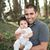# Alok Menghrajani

Previously: security engineer at Square, co-author of HackLang, put the 's' in https at Facebook. Maker of CTFs.

It is common wisdom that NAND and NOR are universal logic gates: any logic circuit can be created using any one of these two gates. This property is called "functional completeness".

But are these the only two (binary) gates with this property? This wikipedia page states there aren't any others:

In digital electronics terminology, the binary NAND gate and the binary NOR gate are the only binary universal logic gates.

Given two signals, A and B, there are 4 possible cominations: AB, A̅B, AB̅, A̅B̅. This implies there are 16 possible binary gates. Some like AND, OR, XOR, NAND, NOR have names. There are 5 gates that don't have any names, so we'll just number them G1-G5:

Let's assume that a gate is universal if it can be used as to create a NOT and an AND gate. As long as we agree that NAND is a universal gate, we don't need to get into too much formalisme

Using G1, you can create a NOT by using the 1 constant. Constants always exist in a circuit, how else can a gate invert a 0 into a 1?

• NOT: 1 G1 A => A̅
• AND: A G1 (1 G1 B) => A & B

G2 is the mirror of G1.

• NOT: A G2 1 => A̅
• AND: (A G2 1) G2 B => A & B

In a similar way, you can use the 0 constant with G4.

• NOT: 0 G4 A => A̅
• AND: 0 G4 ((0 G4 A) G4 B) => A & B

And G5 is the mirror of G4.

• NOT: A G5 0 => A̅
• AND: (A (B G5 0)) G5 0 => A & B

Notice how G1, G2, G5 and G4 form a pattern on the 16 possible gates?